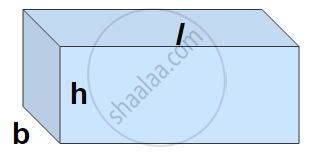# Volume of a Cuboid

#### formula

• Volume of a Cuboid = l × b × h

# Volume of a Cuboid:Volume of a Cuboid = Measure of the space occupied by the cuboid.

The area of the plane region occupied by each rectangle × height.

Volume of a Cuboid = base area × height = length × breadth × height.

Volume of a Cuboid = l × b × h, where l, b, and h are respectively the length, breadth, and height of the cuboid.

#### Example

Find the height of a cuboid whose volume is 275 cm3 and the base area is 25 cm2.
Volume of a cuboid = Base area × Height
Hence height of the cuboid = "Volume of cuboid"/"Base area"
=275/25
= 11 cm
Height of the cuboid is 11 cm.

#### Example

A godown is in the form of a cuboid of measures 60 m × 40 m × 30 m. How many cuboidal boxes can be stored in it if the volume of one box is 0.8 m3?
Volume of one box = 0.8 m3
Volume of godown = 60 × 40 × 30 = 72000 m3
Number of boxes that can be stored in the godown = "Volume of the godown"/"Volume of one box"
= (60 xx 40 xx 30)/(0.8)
= 90,000
Hence the number of cuboidal boxes that can be stored in the godown is 90,000.
If you would like to contribute notes or other learning material, please submit them using the button below.

### Shaalaa.com

Problems on Mensuration - Part 2 [00:10:48]
S
0%